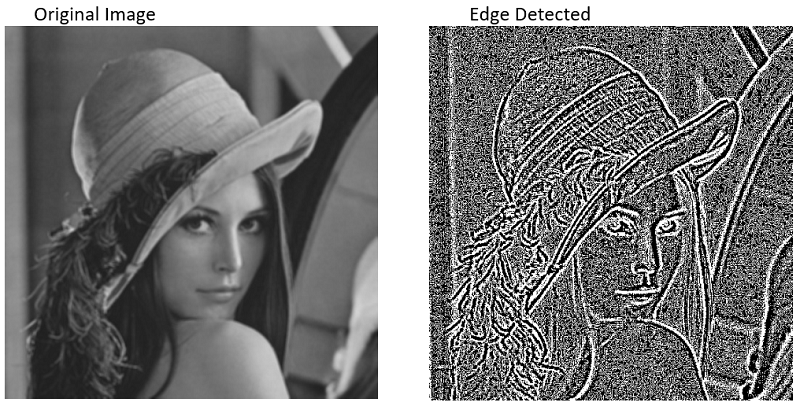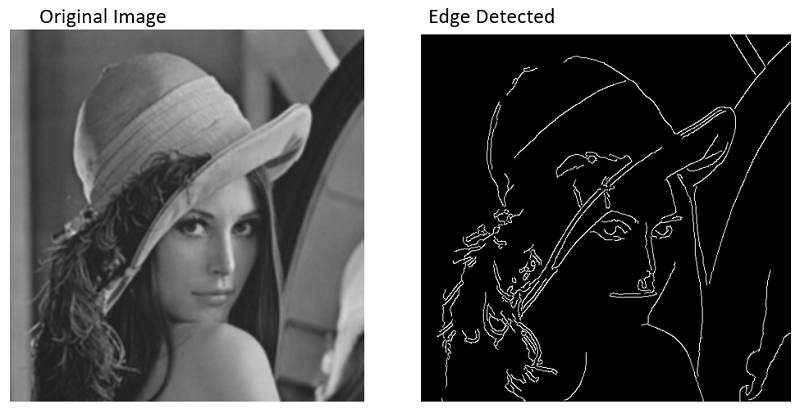# Edge Detection of Image using OpenCV (CV2) in Python

Python | Edge Detection: Here, we will see how we can detect the edge of an image using OpenCv(CV2) in Python?
Submitted by Abhinav Gangrade, on June 20, 2020

Modules used:

For this, we will use the opencv-python module which provides us various functions to work on images.

```General Way:
pip install opencv-python

Pycharm Users:
Go to the project Interpreter and install this module from there.
```

opencv-python Module:

opencv-python is a python library that will solve the Computer Vision Problems and provides us various functions to edit the Images.

Note: The edge Detection is possible only in grayscale Image.

What we will do in this script?

To detect the edges of the images we will use opencv-python various Functions and Provide thresholds.

In this article we will detect the edge of the Image with the help of various functions and the accuracy of edge increases as we go down,

• Sobel Function: This Function will create the Horizontal and vertical edges and after that, we will use the Bitwise or operator to combine them
• Laplacian Function: This Function is the simplest Function in which we just have to put the Grayscale Variable into it, and we will get the edge detected image.
• Canny Function: This is the most powerful function for edge detection and most accurate.

Let's see the code:

## 1) Using Sobel Function

```# importing the module
import cv2

# read the image and store the data in a variable

# make it grayscale
Gray=cv2.cvtColor(image,cv2.COLOR_BGR2GRAY)

# Make it with the help of sobel
# make the sobel_horizontal
# For horizontal x axis=1 and yaxis=0
# for vertical x axis=0 and y axis=1
Horizontal=cv2.Sobel(Gray,0,1,0,cv2.CV_64F)

# the thresholds are like
# (variable,0,<x axis>,<y axis>,cv2.CV_64F)
Vertical=cv2.Sobel(Gray,0,0,1,cv2.CV_64F)

# DO the Bitwise operation
Bitwise_Or=cv2.bitwise_or(Horizontal,Vertical)

# Show the Edged Image
cv2.imshow("Sobel Image",Bitwise_Or)
cv2.imshow("Original Image",Gray)
cv2.waitKey(0)
cv2.destroyAllWindows()
```

Output:## 2) Laplacian Function

```# importing the module
import cv2

# read the image and store the data in a variable

# make it grayscale
Gray=cv2.cvtColor(image,cv2.COLOR_BGR2GRAY)

# Make Laplacian Function
Lappy=cv2.Laplacian(Gray,cv2.CV_64F)

cv2.imshow("Laplacian",Lappy)
cv2.imshow("Original",Gray)
cv2.waitKey(0)
cv2.destroyAllWindows()
```

Output:## 3) Using Canny Function

```# importing the module
import cv2

# read the image and store the data in a variable

# make it grayscale
Gray=cv2.cvtColor(image,cv2.COLOR_BGR2GRAY)

# Make canny Function
canny=cv2.Canny(Gray,40,140)

# the threshold is varies bw 0 and 255
cv2.imshow("Canny",canny)
cv2.imshow("Original",Gray)
cv2.waitKey(0)
cv2.destroyAllWindows()
```

Output:Preparation